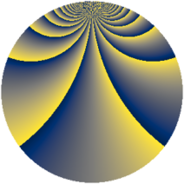# Properties

 Label 2850.2.cmLevel $2850$ Weight $2$ Character orbit 2850.cm Rep. character $\chi_{2850}(29,\cdot)$ Character field $\Q(\zeta_{90})$ Dimension $4800$ Sturm bound $1200$

# Learn more about

## Defining parameters

 Level: $$N$$ $$=$$ $$2850 = 2 \cdot 3 \cdot 5^{2} \cdot 19$$ Weight: $$k$$ $$=$$ $$2$$ Character orbit: $$[\chi]$$ $$=$$ 2850.cm (of order $$90$$ and degree $$24$$) Character conductor: $$\operatorname{cond}(\chi)$$ $$=$$ $$1425$$ Character field: $$\Q(\zeta_{90})$$ Sturm bound: $$1200$$

## Dimensions

The following table gives the dimensions of various subspaces of $$M_{2}(2850, [\chi])$$.

Total New Old
Modular forms 14592 4800 9792
Cusp forms 14208 4800 9408
Eisenstein series 384 0 384

## Trace form

 $$4800q + O(q^{10})$$ $$4800q - 30q^{15} - 60q^{22} + 108q^{25} + 54q^{45} - 108q^{46} + 2304q^{49} + 120q^{51} - 36q^{54} - 60q^{55} - 6q^{60} - 144q^{61} - 600q^{64} + 96q^{66} + 96q^{81} - 162q^{84} - 72q^{85} + 36q^{90} + 192q^{99} + O(q^{100})$$

## Decomposition of $$S_{2}^{\mathrm{new}}(2850, [\chi])$$ into newform subspaces

The newforms in this space have not yet been added to the LMFDB.

## Decomposition of $$S_{2}^{\mathrm{old}}(2850, [\chi])$$ into lower level spaces

$$S_{2}^{\mathrm{old}}(2850, [\chi]) \cong$$ $$S_{2}^{\mathrm{new}}(1425, [\chi])$$$$^{\oplus 2}$$